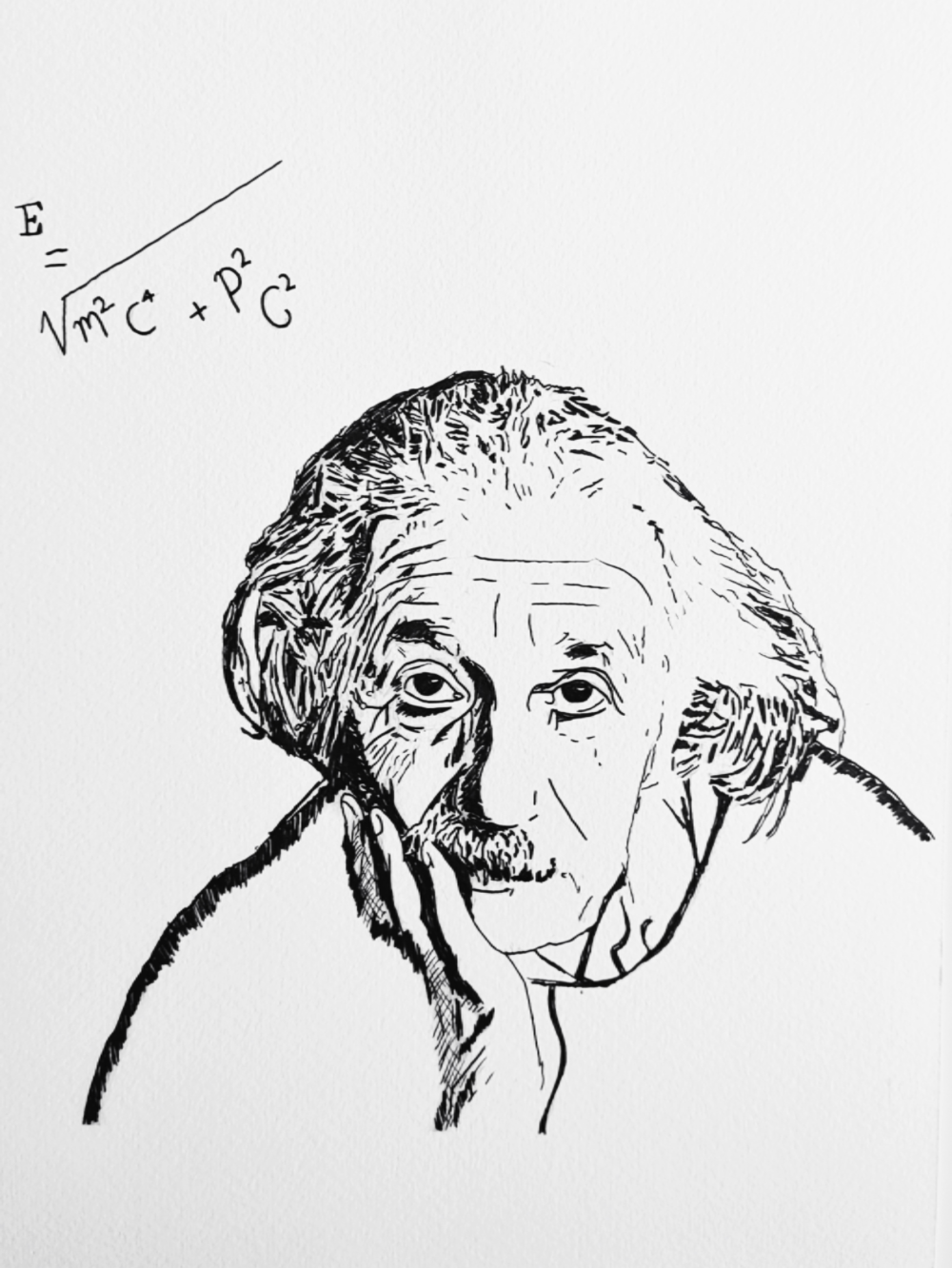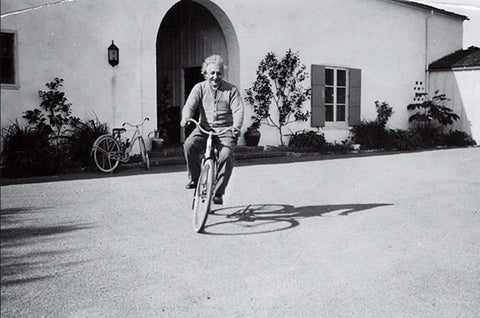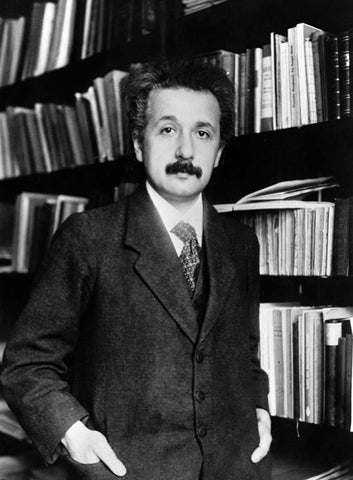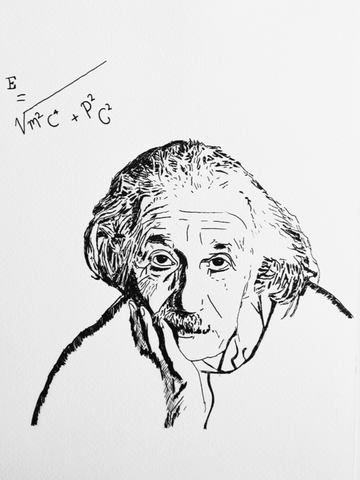# What You Need to Know About Albert Einstein and His Famous Equation

On this day in 1905, Albert Einstein's paper "Does the Inertia of a Body Depend on Its Energy Content?" is published in the physics journal Annalen der Physik, introducing the equation E=mc2. In response to problems with Newtonian mechanics, he developed his special theory of relativity. E = mc2, his mass-energy equivalence formula, has been dubbed "the world's most famous equation."You’ve probably heard of Albert Einstein and his famous equation E=mc^2. You may also know that he was a theoretical physicist who developed the theory of general relativity, which explains how gravity acts as a curvature of space-time. However, there is much more to this brilliant scientist than what you might have read in your high school textbooks or seen in movie biographies. Einstein was born on March 14, 1879 in Ulm, Germany. He excelled in math during his early school years and went on to become a patent clerk, university professor and physicist. His work led to the publication of more than 200 scientific articles and universal recognition as one of the most brilliant minds in history.## What does E=mc^2 mean?

In short, Einstein’s famous equation describes how energy and mass are related. It says that mass and energy are actually the same thing. That might sound confusing at first, but it’s actually a very simple equation that describes how light and matter are actually in the same family. E stands for energy, and m stands for mass. The “c” is the speed of light, which is a really, really fast thing. When you put these letters together and solve for m, you get “the amount of energy needed to create one gram of matter.”

## Why is Einstein’s equation so important?

In short, Einstein’s equation is the reason that nuclear power exists. We can use nuclear energy to create electricity and fuel for submarines, aeroplanes and other vehicles.

It states that the energy (E) in a system (atom, person, solar system) is equal to the total mass (m) multiplied by the square of the speed of light (c, equal to 186,000 miles per second).

The fact that Einstein's equation is so fundamentally important alone made it famous. However, the atomic bomb, one of the most lethal weapons ever created by humans, is largely responsible for its fame.## What does general relativity say about our universe?

Einstein’s theory of general relativity says that the universe is curved. In fact, it’s a giant ball that’s being warped by the gravity of itself and other objects. This means that if you were to fly in a straight line in a spaceship, you’d actually be curving downward. This is because the universe is curved, and your path is actually a curve. General relativity also has a lot to do with time. Einstein’s theory says that time is actually relative and that everything is happening at the same time. What this means is that if you were to travel far enough into space, you could come back and find that less time has passed on Earth than the amount of time you spent travelling. This is because time is relative and changes based on how fast you’re going.

## Where can we find evidence of Einstein’s theory?

You can find evidence of Einstein’s theory pretty much everywhere. You can see it in the way that our galaxy is spinning and how other galaxies are moving away from us. You can also see evidence of Einstein’s theory in the way that light bends around massive objects like the sun and Earth. You can even see evidence in the fact that our planet is not perfectly round but is actually more like an egg shape.

Einstein emerged as the most significant physicist of the 20th century and received the Nobel Prize in Physics in 1921.

Available as an A5 print here

This weeks sketch...